Volume 4, Issue 5-1, October 2015, Pages: 30-39

Variability in Fineness Parameter of Agave Americana L. Fiber "Intra-Plant Study"

Asma El Oudiani1, Slah Msahli1, Faouzi Sakli1, Jean Yves Drean2

1Laboratory of Textile Engineering, University of Monastir, ISET Ksar Hellal, B.P 68 Ksar Hellal, Tunisia

2Laboratory of Textile Physics and Mechanics, ENSITM, 11 rue Alfred Werner, Mulhouse Cedex, France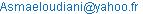(A. E. Oudiani)

Asma El Oudiani, Slah Msahli, Faouzi Sakli, Jean Yves Drean. Variability in Fineness Parameter of Agave Americana L. Fiber "Intra-Plant Study". Advances in Materials. Special Issue: New Methods of Extraction and Characterization of Plant Fibers. Vol. 4, No. 5-1, 2015, pp. 30-39. doi: 10.11648/j.am.s.2015040501.14

Abstract: The aim of this paper is to study the botanical causes (age of plant, maturity of fibers) leading to the variability in fineness parameter for plant fibers, particularly agave Americana L. fiber. The Design of experiment DoE techniques, and other statistical analyses are used by varying the factors of interest in a full factorial design to assess the main effects and the interactions of some factors influencing the fiber fineness. In this work, which represents an intra plant study, fibers are taken from different parts of a same plant, and many controlling factors were considered namely the leaf age (position of the leaf in the plant) and the fiber maturity (position of fibers within the leaf). The output parameters are the fineness and the mean ‘equivalent’ diameter.The main results show that fibers taken from different leaves at the same level of a plant have approximately much close properties. However, fibers located at different positions in the leaf itself (tip, middle or base) exhibit different properties. Besides, fibers belonging to inferior levels of the plant (oldest leaves) have different properties compared to those selected from the top of the plant (youngest leaves). The 2-order interactions have negligible effect on fineness and diameter parameters.

Contents

1. Introduction

Fineness is a main characteristic for textile fibers. However, the definition and the measurement of this property for agave fibers may cause certain shortcoming due to their particular structure. In fact, unlike cotton, wool and synthetic fibers, which are in the form of individual fibers easily separable and with well defined section, agave fibers are rather in form of technical fibers. These technical fibers are composed of elementary (ultimate) fibers sticked together by gums, lignin and non cellulosic materials with unequal proportions along the fiber. Thus, the section form of agave fiber is hard to be defined and measured. To measure quantitatively the fineness of agave fiber, many techniques may be used. For a sample of 100 fibers randomly taken from different plants and leaves, the calculation of fineness (by the gravimetric method) revealed the following results .

Ÿ Mean value: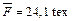Ÿ Coefficient of variation: CV% = 18.32%

Ÿ Measuring error limit: LPE% (95%) = 3,63%

Ÿ Confidence interval: IC (95%) = ± 0.87 tex

Despite the low value of LPE % (inferior to 5 %), the fineness coefficient of variation exhibits a high value. This is to emphasize the great dispersion of results in fineness parameter for agave fiber. Many reasons could explain this dispersion:

i.        Technical fibers are formed of individual (ultimate) fibers sticked together by gums and non cellulosic materials. These fibers haven’t necessarily the same dimensions and they are not regularly arranged all along technical fibers.

ii.        The second reason to explain the dispersion of results may be due to the nature of the fiber itself since it presents a composite structure with unequal proportions of cellulosic and non cellulosic materials (lignin, pectin, gums…).

iii.         Another hypothesis may be raised assuming that the great variability of results may be related to the leaves and fibers collection mode. In fact, fibers can be randomly extracted from different parts of the plant and of the leaf.

To support the last hypothesis, we find it necessary, in the present work, to implement a full experimental design aiming to study the variability in fineness parameter when fibers are taken from different parts of a same plant (intra plant study). In this work, many controlling factors were considered namely the leaf age (position of the leaf in the plant) and the fiber maturity (position of fibers within the leaf). The output parameters are the fineness and the mean ‘equivalent’ diameter.

2. Materials and Methods

2.1. Sampling Plan

Ÿ Leaves collecting plan

In the following part, we describe the mode of collection of leaves from the plant for the intra-plant (in the same plant) study.

Ÿ Number of plants: 1

Ÿ Number of levels : 10 levels of leaves useable

3 levels of falling leaves

the levels of small leaves having a low fiber proportion

Ÿ Number of leaves per level : 2

Ÿ Not collected : one in two leaves

Ÿ Length cut : 20 cm from the base

Agave plant grows in the form of a dense rosette composed of long thick leaves. These leaves are disposed in a spiral defining a line drawn onto the plant stalk and holding the different points of intersection of consecutive leaves . Considering this arrangement illustrated in the scheme of figure 1(a), we have defined the controlling factor of level as one turn of the spiral. The photo of figure 1(b) illustrates the arrangement of leaves at the same level.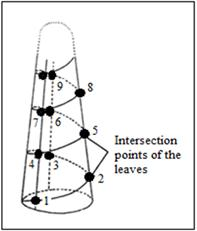(a) Scheme of the spiral creating the leaves(b) Arrangement of leaves in agave plant

Figure 1. Level factor in the plant.

Table 1. Number of collected leaves according to the factor ‘level’

 Level Number of collected leaves Observations 1 0 à Falling leaves, not useable 2 0 à Falling leaves, not useable 3 0 à Falling leaves, not useable 4 2 5 2 6 2 7 2 8 2 9 2 10 2 11 2 12 2 13 2 14 0 à very young leaf (pratically no fibers) Total 20

The levels indicate about the age of the plant. In this manner, for a plant of 14 levels, those at the bottom of the plant (1, 2 and 3) are composed of falling or dead leaves not useable, and it is the same with level (14) in the top of the plant which include very young leaves, practically without fibers.

Thus, for the factor ‘level’, we have considered only the levels where the leaves are useable (not falling), namely the levels from 4 to 13, and in the following parts, the level N=1 corresponds to the fourth level of plant collecting. From each level, we have taken two leaves to work on, so that the total number of leaves is 20.

Ÿ Fiber extraction

The fiber extraction from leaves has been carried out by degrading the leaf parenchyma in seawater.

The period of extraction depends on the level of the leaf as shown in table 2:

Table 2. Period of fibers extraction.

 Level Period (month) 10 – 11 – 12 – 13 2 Others 7

After retrieving from seawater, fibers were rinsed by distilled water, dried at ambient temperature, and then manually individualized. Before testing, fibers were preconditioned as required in the fiber test standard, at a temperature of 20°C ± 2°C and a relative humidity of 65% ± 2%.

Ÿ Fibers sampling plan

The observation of the extracted fibers revealed a significant variation of the diameter between the tip of the leaf and its root, the reason for which we have introduced another controlling factor in the experimental design. This latter presents the fiber position in the leaf defined by three different levels as illustrated in figure 2.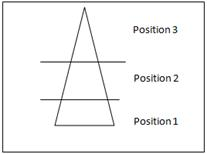Figure 2. Leaf schematization (position factor).

In this way, the experimental design is defined by three controlling factors with their different levels.

- N = level (of leaves in the plant) 1, 2, 3, 4, 5, 6, 7, 8, 9, 10

- F = leaf (from the same level) 1, 2

- P = Position (of fibers in the leaf) 1, 2, 3

All fibers are taken from the same plant (intra plant).

The choice of those factors with their different levels leads to a full factorial experimental design of type 10´2´3 , and containing 60 rows (experiments). This experimental plan was repeated in this study 20 times, and therefore, we have used in total 1200 experiments.

Fibers samples are identified as follows:

N (level from 1 to 10), F (leaf from 1 to 2), P (position from 1 to 3)

For instance, the sample coded 111, represents the first bundle of fibers extracted from position 1 of the leaf 1 (bottom part of leaf 1) itself taken from the first level of the plant (base).

2.2. Fineness Assessment

To measure quantitatively the fineness of agave fiber, many techniques may be used:

Ÿ Gravimetric method

As described in the French standard NF G 07-007 , this method is based on the measurement of fiber weight and length. The linear density, often called ‘fineness’ is determined (in tex) by the following equation:

T (tex) =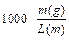This technique requires a microbalance with a precision of at least 1%, and another instrument to measure the fiber length as specified in the French standard NF G 07-006.

Before test, fibers have to be conditioned in a standard atmosphere (20°C ± 2°C for temperature and 65% ± 2% for relative humidity).

Ÿ Projection microscope measuring

This method is essentially used to determine the diameter of wool fibers as described in French standard .NF G 07-004 : fiber sections with standard length (between 4 and 8 mm) are placed on a specimen slide, and moved with a constant pitch under the objective lense (with magnification x500). For the case of hard fibers (like agave fibers), the magnification of 500 times is high enough to observe entirely the fiber diameter, for that reason, we have used a magnification of 200 times.

Furthermore, we have defined the ‘apparent’ diameter characteristic which corresponds to the mean value of the projected diameters, when the fiber takes all the possible orientations with respect to the projection plan , as illustrated in figure 3.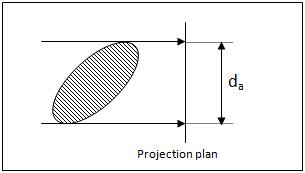Figure 3. Definition of the ‘apparent’ diameter for elliptical cross- section fiber.

The ‘apparent’ diameter is calculated by the following formula:

Da = E (da /A)

With

E (..) : the mathematical expectations operator

E (.. / ..) : the mathematical expectations operator conditional

A = straight section area.

2.3. Statistical Tools

The aim of this study is to define precisely the effect of certain parameters such us the age of the leaf and the position of fibers all along the leaf. An extensive statistical study permit us to demonstrate if the variations of results are assigned only to hazardous fluctuation or to the effect of the input controlling factors considered in this study. This study uses statistical tools such as normality test, response graph, analysis of variance (ANOVA) techniques, developed under Minitab® V14 software.

Ÿ Normality test

Normality tests are used to determine if a data set is well-modeled by a normal distribution and to compute how likely it is for a random variable underlying the data set to be normally distributed   . The hypotheses of this test are:

Null hypothesis = the data follow a normal law

Hypothesis= the data are not normally distributed.

In our work, we have used the probability plot test (Henry plot).

Ÿ Main effects plot

The main effects plot represents the mean value of the output responses for each level of controlling factors. This plot is basically used to compare the significance of controlling factors effects and to determine the most important factor influencing the process.

Ÿ Multivariate analysis

Multivariate analysis (MVA) is based on the statistical principle of multivariate statistics, which involves observation and analysis of more than one statistical outcome variable at a time. In design and analysis, this graphical technique is used to perform the analysis of variance across multiple dimensions while taking into account the effects of all variables (effect of interactions) on the responses .

Ÿ Interaction effects

Interaction effects represent the combined effects of factors on the dependent measure. When an interaction effect is present, the impact of one factor depends on the level of the other factor. Part of the power of ANOVA is the ability to estimate and test interaction effects. As Pedhazur and Schmelkin note, the idea that multiple effects should be studied in research rather than the isolated effects of single variables is one of the important contributions of Sir Ronald Fisher. When interaction effects are present, it means that interpretation of the main effects is incomplete or misleading.

In the digram of interaction effects, parallel Interaction results whose lines do not cross (as in the figure at left) are called "ordinal" interactions. If the slope of lines is not parallel in an ordinal interaction, the interaction effect will be significant, given enough statistical power. If the lines are parallel, then there is no interaction effect .

Ÿ homogeneity of variances test

In some statistical tests, for example the analysis of variance, we assume that variances are equal across groups or samples (homogeneity of variances). The Bartlett test can be used to verify whether the data are normally distributed . Bartlett's test is sensitive to departures from normality. That is, if samples come from non-normal distributions, then Bartlett's test may simply be testing for non-normality. The Levene test  is an alternative to the Bartlett test that is less sensitive to departures from normality.

Ÿ Analysis of variance

The analysis of variance is a statistical test which allows to see if the controlling factor effects are more or less significant on the response. The method is based on the comparison between the real variances of the model coefficients and the variances of these same coefficients if the controlling factors have no effects  .

This test consists on calculating a statistic F from the coefficients of the established model and then to compare it with F values taken from statistical tables of Snedecor law .

Ÿ If Fcal > F1% , the difference is highly significant with 1% risk of error.

Ÿ If F5% < Fcal < F1% the difference is significant with 5% risk of error.

Ÿ If Fcal < F5% the difference is not significant with 5% risk of error.

3. Results and Discussion

Graphics of figures 4, 5, 6 and 7 show the evolution of fineness and diameter as a function of the controlling factors described previously. It is worth noting that those effects are calculated by the mean values of the obtained results when the factor is fixed at a certain level regardless of any other levels and factors.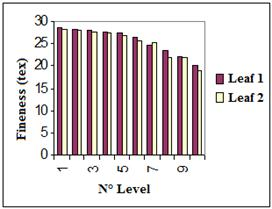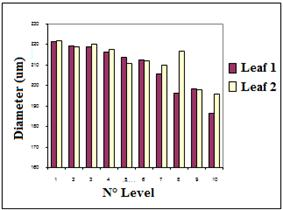Figure 4. Evolution of fineness and diameter as function of factor ‘Leaf’.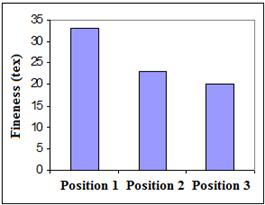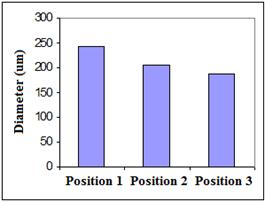Figure 5. Evolution of fineness and diameter as function of factor ‘Position’.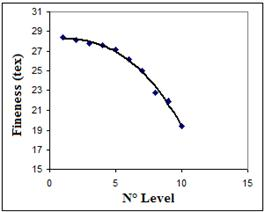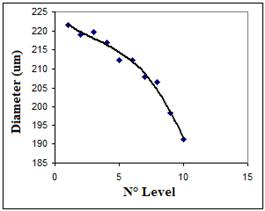Figure 6. Evolution of fineness and diameter as function of factor ‘Level’.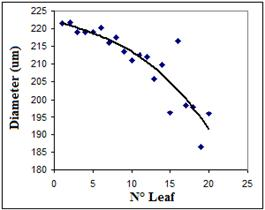Figure 7. Evolution of fineness and diameter as function of factor ‘Leaf position’.

Graphics of figures 5, 6 and 7 show a decrease of fineness and diameter parameters with the three controlling factors.

For the ‘position factor’, which is a priori the most important one, the decrease is more noticeable. This means that the finest fibers are situated in the tip of the leaf. We may explain this phenomenon by the dominance of lignin and parenchyma materials in the bottom part of the leaf, which was demonstrated by many other research works on this fiber class .

Regarding the factor ‘leaf’, we remark (figure 4) a small difference of the fineness parameter between two leaves of the same level. This difference will be statistically evaluated in the following part.

From figure 6, which illustrates the evolution of the parameters with the factor ‘level’, we note a decrease of fineness and diameter parameters for the youngest leaves. This drives us to introduce the notion of ‘biological maturation’ which may explain the observed decrease. As a matter of fact, the reduction of the fineness and the diameter is very significant from the 5th level, which suggests that leaves collected from inferior levels have reached the biological maturation.

Ÿ Normality test

Figure 8 presents the probability plot related to the normal law for both studied characteristics.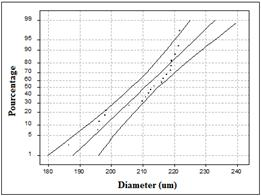a                                                                       b

Figure 8. Probability plot following a normal distribution for: (a) the fineness and (b) the diameter.

The Henry plot (probability plot) coefficients determined from curves of figure 8, are gathered in table 3.

Table 3. Normality test results (Henry plot coefficient).

 Parameter Coefficient of the probability (Henry) plot Fineness 0.933 Diameter 0.940

We notice that Henry plot coefficients have high values approximately equal to 1 (» 1), which means that the experimental data could be assimilated to a normal law. Consequently, all the parameters which may affect those data have the same importance and no one is dominant.

Ÿ Analysis of the main effects

The controlling factors considered in this study are:

N = level (10 modalities)

F = Leaf (2 modalities)

P = Position (3 modalities)

The main effects of those parameters on the output parameters are presented in figures 9 and 10.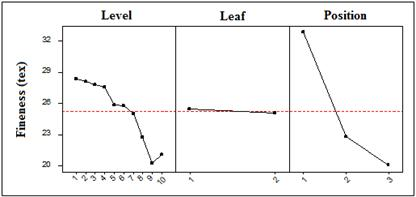Figure 9. Main effects plot for the fineness.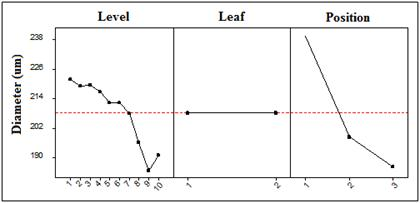Figure 10. Main effects plot for the diameter.

From graphics of figures 9 and 10, we deduce the importance (significance) of each controlling factor presented in table 4.

Table 4. Classification of the main effects of the controlling factors .

 Fineness Diameter Level Leaf Position

With: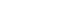Insignificant;Significant;Highly significant

The results of the main effects analysis show that the factor ‘leaf’ is negligible, whereas the factor ‘position’ is the most influential for both studied parameters. The explanation of these more or less important effects is due to the presence of lignin and gums and their effect on the fiber structure.

Ÿ Analysis of interaction effects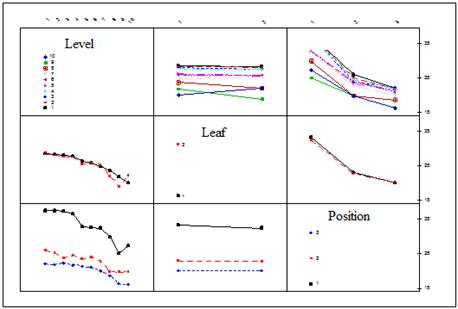Figure 11. Interaction effects relative to fineness.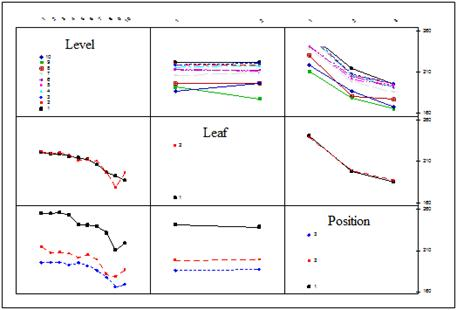Figure 12. Interaction effects relative to diameter.

An interaction effect is present if the graphic of one factor level response depends on the other level factors, which is pictured by non parallel plots. Using plots of figures 11 and 12, we can deduce the table 5 summarizing the presence and the importance of interaction effects between controlling factors.

Table 5. Identification and classification of interaction effects between two factors.

 Fineness Diameter N ´F - - N ´P - - F ´P - -

With : - : the interaction is negligible or absent

´ : the interaction is present and as important as the number of x is raised.

We note that all interactions are negligible for the fineness and diameter parameters.

Ÿ Equality of variances

Levene test results are gathered in table 6:

Table 6. Levene test results for equality of variances.

 Fineness Diameter Statistics 3.28 2.119 Probability 0.0003 0.000 Conclusion Null hypothesis rejected

Ÿ Analysis of variance

The analysis of variance is the most decisive test since it allows us to classify controlling factors and to identify the most important ones. Practically, this involves the choice of leaves from the plant as well as the selection of fibers from the leaf itself.

Results of analysis of variance are presented by multivariate analysis (figures 13 and 14 and tables 7 and 8).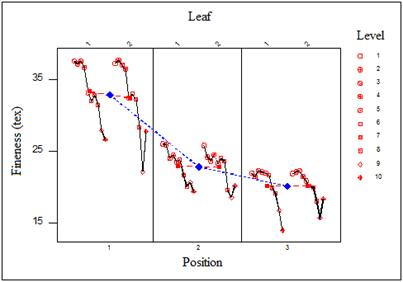Figure 13 . Multivariate analysis relative to fineness.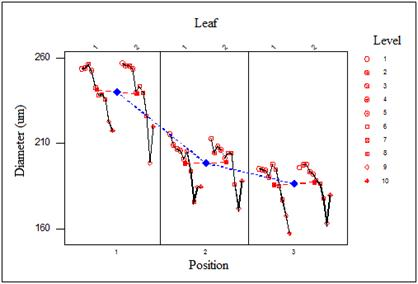Figure 14. Multivariate analysis relative to diameter.

Table 7. Analysis of variance results for fineness.

 DL F F (5%) F (1%) P Conclusion N 9 15.73 1.89 2.42 1.9 E-24 ´´ F 1 0.61 3.85 6.65 0.435 0 P 2 268.78 3.01 4.62 2.3 E -96 ´´ N ´F 9 0.77 1.89 2.42 0.644 0 N ´P 18 1.22 1.62 1.95 0.240 0 F ´P 2 0.32 3.01 4.62 0.728 0 N ´F ´P 18 0.26 1.62 1.95 0.999 0

With:0 : Negligible

´ : Significant

´´ : Highly significant

Table 8. Analysis of variance results for diameter.

 DL F F (5%) F (1%) P Conclusion N 9 17.77 1.89 2.42 8.84 E -28 ´´ F 1 0.00 3.85 6.65 0.956 0 P 2 278.87 3.01 4.62 2.5 E -99 ´´ N ´F 9 0.9 1.89 2.42 0.525 0 N ´P 18 0.55 1.62 1.95 0.933 0 F ´P 2 0.41 3.01 4.62 0.664 0 N ´F ´P 18 0.41 1.62 1.95 0.986 0

Table 9. Recapitulation of ANOVA results.

 Fineness Diameter N F P N ´F N ´P F ´P N ´F ´P

With:Negligible;Significant;Highly significant

Table 9 summarizes the main results of intra plant variability study on fineness and diameter. The assessment of controlling factors effects, permit us practically to orientate the collecting and the choice of fibers for future applications.

The factor ‘leaf’ is negligible for both characteristics. This means that fibers taken from different leaves at the same level of a plant have approximately much close properties. However, the factor ‘position’ is the most crucial one, which means that fibers located at different positions in the leaf (tip, middle or base) exhibit different properties.

Besides, the factor ‘level’ is much important, given that fibers belonging to inferior levels of the plant (oldest leaves) have different properties compared to those selected from the top of the plant (youngest leaves). The 2-order interactions have negligible effect on fineness and diameter parameters. Consequently, the simultaneous changes of factors levels do not alter fiber fineness.

4. Conclusions

In this work, a study of intra plant variability in agave fiber fineness was conducted. It is based on the Design of experiments techniques and statistical analyses. By taking into account the variation of modalities related to the fiber collecting mode, some parameters were defined and considered as controlling factors, namely the position of the fiber in the leaf (fiber maturity) and the level of the leaf in the plant (leaf age).

From the normality test, we found that Henry plot coefficients have high values approximately equal to 1 (» 1), which means that the experimental data could be assimilated to a normal law.

The results of the main effects analysis show that the factor ‘leaf’ is negligible, in other words, fibers taken from different leaves of the same level have approximately much close properties. On the other hand, the factor ‘position’ is the most influential for both studied parameters which means that fibers located at different positions in the leaf (tip, middle or base) exhibit different values of fineness.

The factor ‘level’ is also crucial, given that fibers belonging to inferior levels of the plant (oldest leaves) have different fineness properties compared to those selected from the top of the plant (youngest leaves).

References

1. Msahli Slah, Etude du potentiel textile des fibres d’Agave Americana L., PhD thesis, No. °02Mulh0691, Université de Haute Alsace, Mulhouse, France, 2002.
2. J. P. Lafon & C. Tharavd-Prayer, Biologie des plantes cultivées, Tome 1 : organisation physiologique de la nutrition, Paris : Gille LEVY.
3. M. Vigier, Pratique des plans d’expériences, méthode Taguchi et compléments, Paris : Les éditions d’Organisation, 1991.
4. Essais des fibres – Détermination de la masse linéique (ou titre) des fibres, NF G 07 – 007 (Avril 1961) Paris : AFNOR, 1983, page 272.
5. Essais sur fibres – Détermination du diamètre des fibres de laine – méthode du microscope à projection, NF G 07 – 004 (Décembre 1973), Paris : AFNOR, 1983, page 265.
6. C. Dreyer, Contribution à la modélisation de l’influence des caractéristiques des fibres de laine peignée sur les propriétés des textiles linéaires, Thèse de doctorat, Université de Haute Alsace - Mulhouse, 1994.
7. S. F. Arnold, Mathematical Statistics, Prentice-Hall, 1990, pages 383 – 384.
8. J. J. Filliben, The Probability Plot Correlation Coefficient Test for Normality Technometrics, 1975, Vol. 17, pages 111.
9. R. B. Daugostino & M. A. Stevens, Goodness of Fit Techniques, Marcel Dekker, 1986
10. A. Agreshi, Categorial Data Analysis, John Wiley and Sons, 1990.
11. C. R. Hicks, Fundamental concepts in the design of experiments, 3rd Ed, CBC College Publishing, 1982.
12. M. B. Brown & A. B. Forsythe, Journal of the American Statistical Association, 1974, N° 69, pages 364 – 367.
13. H. Levene, Contributions to Probability and Statistics, Stanford University Press, CA, 1960, pages 278 – 292.
14. G. A. Milliken & D. E. Johnson, Analysis of Messy Data, Vol. 1: Designed Experiments, Van Reinhold, 1984.
15. P. R. Nelson, A comparison of sample seizes for the analysis of means and analysis of variances, Journal of Quality Technology, 1983, N° 15, pages 33 – 39.
16. R. A. Olshen, The conditional level of the F-test, Journal of the American Association, 1973, N° 68, pages 692 – 698.
17. Herbert R. Muerserger, Mathews Textile Fibers, Their Physical, Microscopy and Chemical Properties, 2nd Ed, London: Chapman et Hall, Limited, 1954, pages 360 – 438.

 Contents 1. 2. 2.1. 2.2. 2.3. 3. 4.
Article ToolsAbstractPDF(375K)